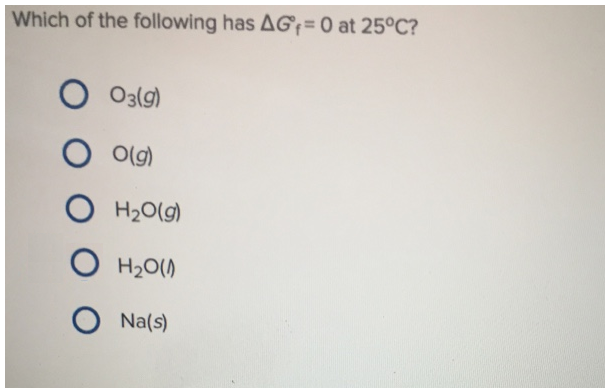# Problem: Which of the following has ΔG°f = 0 at 25°C?a. O3(g)b. O(g)c. H2O(g)d. H2O(l)e. Na(s)

###### FREE Expert Solution

A molecule or atom with ΔG=0 at standard conditions are molecules that is in its natural form. In the

83% (490 ratings)###### Problem Details

Which of the following has ΔG°f = 0 at 25°C?

a. O3(g)

b. O(g)

c. H2O(g)

d. H2O(l)

e. Na(s)